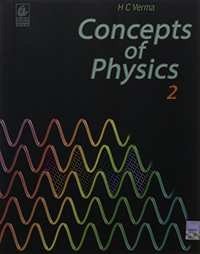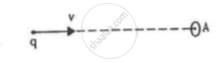CBSE (Science) Class 11CBSE
Share

# H.C. Verma solutions for Class 12 Physics chapter 18 - Electromagnetic Waves

## Concepts of Physics - Vol. 2

#### H.C. Verma Concepts of Physics - Vol. 2## Chapter 18: Electromagnetic Waves

#### Chapter 18: Electromagnetic Waves Exercise Short Answers solutions [Page 338]

Short Answers | Q 1 | Page 338

In a microwave oven, the food is kept in a plastic container and the microwaves is directed towards the food. The food is cooked without melting or igniting the plastic container. Explain.

Short Answers | Q 2 | Page 338

A metal rod is placed along the axis of a solenoid carrying a high-frequency alternating current. It is found that the rod gets heated. Explain why the rod gets heated.

Short Answers | Q 3 | Page 338

Can an electromagnetic wave be deflected by an electric field or a magnetic field?

Short Answers | Q 4 | Page 338

A wire carries an alternating current i = i0 sin ωt. Is there an electric field in the vicinity of the wire?

Short Answers | Q 5 | Page 338

A capacitor is connected to an alternating-current source. Is there a magnetic field between the plates?

Short Answers | Q 6 | Page 338

Can an electromagnetic wave be polarised?

Short Answers | Q 7 | Page 338

A plane electromagnetic wave is passing through a region. Consider (a) electric field (b) magnetic field (c) electrical energy in a small volume and (d) magnetic energy in a small volume. Construct the pairs of the quantities that oscillate with equal frequencies.

#### Chapter 18: Electromagnetic Waves Exercise MCQ solutions [Pages 338 - 339]

MCQ | Q 1 | Page 338

A magnetic field can be produced by

• a moving charge

• a changing electric field

• Neither of them

• Both of them

MCQ | Q 2 | Page 338

A compass needle is placed in the gap of a parallel plate capacitor. The capacitor is connected to a battery through a resistance. The compass needle

• does not deflect

• deflects for a very short time and then comes back to the original position

• deflects and remains deflected as long as the battery is connected

• deflects and gradually comes to the original position in a time that is large compared to the time constant

MCQ | Q 3 | Page 338

Dimensions of 1/(µ0ϵ0) is

• L/T

• T/L

•  L2/T2

• T2/L2.

MCQ | Q 4 | Page 338

Electromagnetic waves are produced by

• a static charge

• a moving charge

•  an accelerating charge

• chargeless particles

MCQ | Q 5 | Page 338

An electromagnetic wave going through vacuum is described by
E = E0 sin (kx − ωt); B = B0 sin (kx − ωt).
Then,

• E0 k = B0 ω

• E0 B0 = ωk

• E0 ω = B0 k

• None of these

MCQ | Q 6 | Page 339

An electric field vec E and a magnetic field vec B exist in a region. The fields are not perpendicular to each other.

• This is not possible.

• No electromagnetic wave is passing through the region.

• An electromagnetic wave may be passing through the region.

• An electromagnetic wave is certainly passing through the region.

MCQ | Q 7 | Page 339

Consider the following two statements regarding a linearly polarised, plane electromagnetic wave :

(A) The electric field and the magnetic field have equal average values.
(B) The electric energy and the magnetic energy have equal average values.

• Both A and B are true.

• A is false but B is true.

• B is false but A is true

• Both A and B are false.

MCQ | Q 8 | Page 339

A free electron is placed in the path of a plane electromagnetic wave. The electron will start moving

• along the electric field

• along the magnetic field

• along the direction of propagation of the wave

• in a plane containing the magnetic field and the direction of propagation

MCQ | Q 9 | Page 339

A plane electromagnetic wave is incident on a material surface. The wave delivers momentum p and energy E.

• p = 0, E ≠ 0

• p ≠ 0, E = 0

• p ≠ 0, E ≠ 0

• p = 0, E = 0

#### Chapter 18: Electromagnetic Waves Exercise MCQ solutions [Page 339]

MCQ | Q 1 | Page 339

An electromagnetic wave going through vacuum is described by
E = E0 sin (kx − ωt).
Which of the following is/are independent of the wavelength?

• k

• ω

• k/ω

MCQ | Q 2 | Page 339

Displacement current goes through the gap between the plates of a capacitor when the charge of the capacitor

(a) increases
(b) decreases
(c) does not change
(d) is zero

MCQ | Q 3 | Page 339

Speed of electromagnetic waves is the same

• for all wavelengths

• in all media

• for all intensities

• for all frequencies

MCQ | Q 4 | Page 339

Which of the following have zero average value in a plane electromagnetic wave?
(a) Electric field
(b) Magnetic field
(c) Electric energy
(d) Magnetic energy

MCQ | Q 5 | Page 339

The energy contained in a small volume through which an electromagnetic wave is passing oscillates with

• zero frequency

•  the frequency of the wave

• half the frequency of the wave

• double the frequency of the wave

#### Chapter 18: Electromagnetic Waves solutions [Pages 339 - 399]

Q 1 | Page 339

Show that the dimensions of the displacement current ∈_0 (d varphi_E)/dt are that of an electric current .

Q 2 | Page 339

A point charge is moving along a straight line with a constant velocity v. Consider a small area A perpendicular to the motion of the charge. Calculate the displacement current through the area when its distance from the charge is x. The value of x is not large, so that the electric field at any instant is essentially given by Coulomb's law.Q 3 | Page 399

A parallel-plate capacitor of plate-area A and plate separation d is joined to a battery of emf ε and internal resistance R at t = 0. Consider a plane surface of area A/2, parallel to the plates and situated symmetrically between them. Find the displacement current through this surface as a function of time.

Q 4 | Page 339

Consider the situation of the previous problem. Define displacement resistance Rd = V/idof the space between the plates, where V is the potential difference between the plates and id is the displacement current. Show that Rd varies with time as R_d = R(e^(t"/"tau) - 1) .

Q 5 | Page 339

Using B = µ0 H, find the ratio E0/H0 for a plane electromagnetic wave propagating through vacuum. Show that it has the dimensions of electric resistance. This ratio is a universal constant called the impedance of free space.

Q 6 | Page 339

The sunlight reaching Earth has maximum electric field of 810 Vm−1. What is the maximum magnetic field in this light?

Q 7 | Page 339

The magnetic field in a plane electromagnetic wave is given by
B = (200 µT) sin [(4.0 × 1015s−1)(t−x/c)].
Find the maximum electric field and the average energy density corresponding to the electric field.

Q 8 | Page 339

A laser beam has intensity 2.5 × 1014 W m−2. Find amplitudes of electric and magnetic fields in the beam.

Q 9 | Page 339

The intensity of the sunlight reaching Earth is 1380 W m−2. Assume this light to be a plane, monochromatic wave. Find the amplitudes of electric and magnetic fields in this wave.

## Chapter 18: Electromagnetic Waves

#### H.C. Verma Concepts of Physics - Vol. 2## H.C. Verma solutions for Class 12 Physics chapter 18 - Electromagnetic Waves

H.C. Verma solutions for Class 12 Physics chapter 18 (Electromagnetic Waves) include all questions with solution and detail explanation. This will clear students doubts about any question and improve application skills while preparing for board exams. The detailed, step-by-step solutions will help you understand the concepts better and clear your confusions, if any. Shaalaa.com has the CBSE Concepts of Physics - Vol. 2 solutions in a manner that help students grasp basic concepts better and faster.

Further, we at Shaalaa.com provide such solutions so that students can prepare for written exams. H.C. Verma textbook solutions can be a core help for self-study and acts as a perfect self-help guidance for students.

Concepts covered in Class 12 Physics chapter 18 Electromagnetic Waves are Elementary Facts About Electromagnetic Wave Uses, Electromagnetic Spectrum, Transverse Nature of Electromagnetic Waves, Electromagnetic Waves, Displacement Current.

Using H.C. Verma Class 12 solutions Electromagnetic Waves exercise by students are an easy way to prepare for the exams, as they involve solutions arranged chapter-wise also page wise. The questions involved in H.C. Verma Solutions are important questions that can be asked in the final exam. Maximum students of CBSE Class 12 prefer H.C. Verma Textbook Solutions to score more in exam.

Get the free view of chapter 18 Electromagnetic Waves Class 12 extra questions for Physics and can use Shaalaa.com to keep it handy for your exam preparation

S